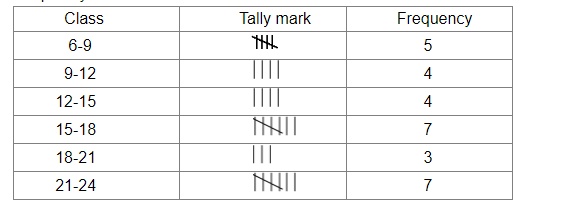# Construct a frequency table for the following ages (in years) of 30 students using equal class intervals, one of them being 9−12, where 12 is not included.

Question:

Construct a frequency table for the following ages (in years) of 30 students using equal class intervals, one of them being 912, where 12 is not included.
18,12,7,6,11,15,21,9,8,13,15,17,22,19,14,21,23,8,12,17,15,6,18,23,22,16,9,21,11,16.

Solution:

The minimum observation is 6 and the maximum observation is 24.
Therefore, classes of the same size covering the given data are 6-9, 9-12, 12-15, 15-18, 18-21 and 21-24.
Frequency distribution table: